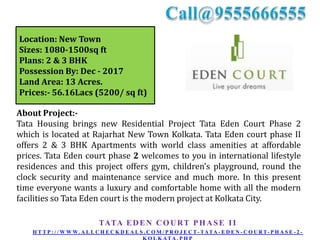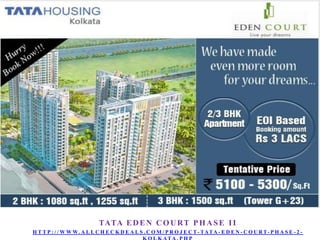Successfully reported this slideshow.

# Tata Eden Court Phase 2 – New Housing Project at Kolkata Call 9555666555

4

Share×
1 of 21
1 of 21

# Tata Eden Court Phase 2 – New Housing Project at Kolkata Call 9555666555

4

Share

Tata Housing brings new Residential Project Tata Eden Court Phase 2 which is located at Rajarhat New Town Kolkata.
Tata Eden Court Phase 2
Call @ 9555666555
http://www.allcheckdeals.com/project-tata-eden-court-phase-2-kolkata.php

Tata Housing brings new Residential Project Tata Eden Court Phase 2 which is located at Rajarhat New Town Kolkata.
Tata Eden Court Phase 2
Call @ 9555666555
http://www.allcheckdeals.com/project-tata-eden-court-phase-2-kolkata.php

## More Related Content

### Related Books

Free with a 14 day trial from Scribd

See all

### Related Audiobooks

Free with a 14 day trial from Scribd

See all

### Tata Eden Court Phase 2 – New Housing Project at Kolkata Call 9555666555

1. 1. Tata Eden Court Phase II TATA E D E N C O U R T P H A S E I I H T T P : / / W W W. A L L C H E C K D E A L S . C O M / P R O J E C T - TATA - E D E N - C O U R T- P H A S E - 2 -
2. 2. Location: New Town Sizes: 1080-1500sq ft Plans: 2 & 3 BHK Possession By: Dec - 2017 Land Area: 13 Acres. Prices:- 56.16Lacs (5200/ sq ft) About Project:Tata Housing brings new Residential Project Tata Eden Court Phase 2 which is located at Rajarhat New Town Kolkata. Tata Eden court phase II offers 2 & 3 BHK Apartments with world class amenities at affordable prices. Tata Eden court phase 2 welcomes to you in international lifestyle residences and this project offers gym, children’s playground, round the clock security and maintenance service and much more. In this present time everyone wants a luxury and comfortable home with all the modern facilities so Tata Eden court is the modern project at Kolkata City. TATA E D E N C O U R T P H A S E I I H T T P : / / W W W. A L L C H E C K D E A L S . C O M / P R O J E C T - TATA - E D E N - C O U R T- P H A S E - 2 -
3. 3. TATA E D E N C O U R T P H A S E I I H T T P : / / W W W. A L L C H E C K D E A L S . C O M / P R O J E C T - TATA - E D E N - C O U R T- P H A S E - 2 -
4. 4. TATA E D E N C O U R T P H A S E I I H T T P : / / W W W. A L L C H E C K D E A L S . C O M / P R O J E C T - TATA - E D E N - C O U R T- P H A S E - 2 -
5. 5. About Developer:TATA Housing Development Company Ltd. established in 1984 is a fully owned subsidiary of TATA Sons Limited. TATA Housing has pioneered the concept of property development and has stood out in the industry with its ethical business practices, rapidly acquiring a public image as a Quality Conscious And Reliable Developer. We believe in creating a home or an office based on an understanding of consumer needs and preferences. Hence, property is developed only after market research to determine the consumer needs and the project is designed and implemented according to consumer preferences. TATA E D E N C O U R T P H A S E I I H T T P : / / W W W. A L L C H E C K D E A L S . C O M / P R O J E C T - TATA - E D E N - C O U R T- P H A S E - 2 -
6. 6. TATA E D E N C O U R T P H A S E I I H T T P : / / W W W. A L L C H E C K D E A L S . C O M / P R O J E C T - TATA - E D E N - C O U R T- P H A S E - 2 -
7. 7. Tata Eden Court Phase II Type Size INR Classification 2BR-2T (Small) 1080 sq ft 56.16Lacs (5200/ sq ft) APARTMENT 2BR-2T (Large) 1255 sq ft 65.26Lacs (5200/ sq ft) APARTMENT 3BR-3T (Small) 1500 sq ft 78Lacs (5200/ sq ft) APARTMENT TATA E D E N C O U R T P H A S E I I H T T P : / / W W W. A L L C H E C K D E A L S . C O M / P R O J E C T - TATA - E D E N - C O U R T- P H A S E - 2 -
8. 8. Tata Eden Court Phase II 2BR-2T (Small) TATA E D E N C O U R T P H A S E I I H T T P : / / W W W. A L L C H E C K D E A L S . C O M / P R O J E C T - TATA - E D E N - C O U R T- P H A S E - 2 -
9. 9. Tata Eden Court Phase II 2BR-2T (Large) TATA E D E N C O U R T P H A S E I I H T T P : / / W W W. A L L C H E C K D E A L S . C O M / P R O J E C T - TATA - E D E N - C O U R T- P H A S E - 2 -
10. 10. Tata Eden Court Phase II 3BR-3T (Small) TATA E D E N C O U R T P H A S E I I H T T P : / / W W W. A L L C H E C K D E A L S . C O M / P R O J E C T - TATA - E D E N - C O U R T- P H A S E - 2 -
11. 11. TATA E D E N C O U R T P H A S E I I H T T P : / / W W W. A L L C H E C K D E A L S . C O M / P R O J E C T - TATA - E D E N - C O U R T- P H A S E - 2 -
12. 12. Location Plan TATA E D E N C O U R T P H A S E I I H T T P : / / W W W. A L L C H E C K D E A L S . C O M / P R O J E C T - TATA - E D E N - C O U R T- P H A S E - 2 -
13. 13. TATA E D E N C O U R T P H A S E I I H T T P : / / W W W. A L L C H E C K D E A L S . C O M / P R O J E C T - TATA - E D E N - C O U R T- P H A S E - 2 -
14. 14. TATA E D E N C O U R T P H A S E I I H T T P : / / W W W. A L L C H E C K D E A L S . C O M / P R O J E C T - TATA - E D E N - C O U R T- P H A S E - 2 -
15. 15. TATA E D E N C O U R T P H A S E I I H T T P : / / W W W. A L L C H E C K D E A L S . C O M / P R O J E C T - TATA - E D E N - C O U R T- P H A S E - 2 -
16. 16. TATA E D E N C O U R T P H A S E I I H T T P : / / W W W. A L L C H E C K D E A L S . C O M / P R O J E C T - TATA - E D E N - C O U R T- P H A S E - 2 -
17. 17. TATA E D E N C O U R T P H A S E I I H T T P : / / W W W. A L L C H E C K D E A L S . C O M / P R O J E C T - TATA - E D E N - C O U R T- P H A S E - 2 -
18. 18. TATA E D E N C O U R T P H A S E I I H T T P : / / W W W. A L L C H E C K D E A L S . C O M / P R O J E C T - TATA - E D E N - C O U R T- P H A S E - 2 -
19. 19. TATA E D E N C O U R T P H A S E I I H T T P : / / W W W. A L L C H E C K D E A L S . C O M / P R O J E C T - TATA - E D E N - C O U R T- P H A S E - 2 -
20. 20. TATA E D E N C O U R T P H A S E I I H T T P : / / W W W. A L L C H E C K D E A L S . C O M / P R O J E C T - TATA - E D E N - C O U R T- P H A S E - 2 -
21. 21. THANK YOU ! TATA E D E N C O U R T P H A S E I I H T T P : / / W W W. A L L C H E C K D E A L S . C O M / P R O J E C T - TATA - E D E N - C O U R T- P H A S E - 2 -